# G484- Circular Motion and Oscillations

?
• Created by: Rosie
• Created on: 21-04-14 17:36

x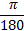1 of 47

x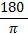2 of 47

the angle subtended at the centre of a circle by an arc of length equal to the circle's radius

3 of 47

## angular displacement equation (circles)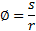s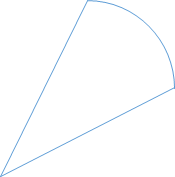r

Ø

4 of 47

mg=TcosØ

5 of 47

## conical pendulum Fc=... (Ø at top)

Fc=TsinØ

(also Fc=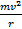)

6 of 47

## Centripetal Force definition

Resultant force, acting towards the centre of a circle, causing an object to move in a circular path

7 of 47

## How does an object move in a circle?

If there is a force acting perpendicular to the velocity, the object moves in a circle

8 of 47

## Centripetal force equation

Fc=(also Fc=mac)

9 of 47

## Centripetal acceleration definition

the acceleration of a body moving in a circle with constant velocity, acting towards the circle's centre

10 of 47

## Centripetal acceleration equation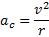11 of 47

## Period definition

time taken for one complete cycle

12 of 47

## Period equation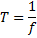(f= cyclic frequency)

13 of 47

## Cyclic frequency definition

number of cycles per unit time

14 of 47

## Cyclic frequency equation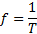also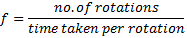15 of 47

## Linear (immediate) velocity equation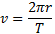16 of 47

## Angular frequency definition

change in angle per unit time

17 of 47

## angular frequency equation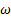=2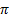f

also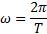18 of 47

Fc=T+mg

19 of 47

Fc=T-mg

20 of 47

## Newton's Law of Gravitation

The gravitational force of attraction between two objects is directly proportional to the product of their masses and inversely proportional to the square of the distance between their centres

21 of 47

## Gravitational force of attraction equation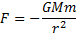22 of 47

## Gravitational field strength definition

the force per unit mass (like acceleration)

(on Earth's surface g=9.81)

23 of 47

## Gravitational field strength equation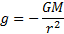24 of 47

## Geostationary orbit definition

an orbit of the earth by a satellite that has the same time period as the rotation of the earth and is in the equatorial plane

25 of 47

## Geostationary satellites must...

• have their orbit centred on the Earth's centre
• be over the equator
• travel from west to east
• have a period of 24hrs equal to the Earth's period of rotation
26 of 47

## Kepler's 3rd Law

The square of the period (T) of a planet is directly proportional to the cube of its separation (r) from the sun

27 of 47

## free oscillation definition

an oscillation with no driving mechanism, no variation in amplitude and no friction (e.g. a swinging clock pendulum)

28 of 47

## displacement definition (SHM)

(x) the distance an object has moved from its equilibrium position

29 of 47

## amplitude definition (SHM)

(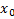, A)

The maximum displacement from the equilibrium position

30 of 47

## Period definition (SHM)

(T,)

Time taken for one complete oscillation
and
is independent from amplitude

31 of 47

## Frequency definition (SHM)

(f,)

number of oscillations per unit time

32 of 47

## angular frequency (SHM)

(, 2f,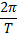)

related to the frequency of the motion and inversely related to the period

33 of 47

## Phase difference definition (SHM)

( Ø )

The fraction of an oscillation that one wave leads/lags behind another

34 of 47

## Simple Harmonic Motion definition

when the acceleration (a) of an object is proportional and in the opposite direction to the displacement (x) from the equilibrium position

(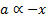)

35 of 47

## eqn. for displacement starting from max displaceme

x=A cos(2ft)

36 of 47

## eqn. for displacement starting from equilibrium

x=A sin(2ft)

37 of 47

## Kinetic + Potential energy at equilibrium

maximum Kinetic energy

0 potential energy

38 of 47

## Kinetic + Potential energy at max. displacement

0 Kinetic energy

maximum Potential energy

39 of 47

## maximum velocity equation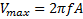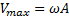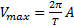40 of 47

## Forced oscillations

occur when an object is forced to vibrate at the frequency of an external source

41 of 47

## Damping

converts kinetic energy into other forms, so reduces the amplitude, and is the result of friction

42 of 47

## Critical damping

the minimum amount of damping needed to cause a system to return to equilibrium without oscillating

43 of 47

## Resonance

the dramatic build up of a large amplitude oscillation when the frequencies of the vibrating objects match

44 of 47

## Resonance (useful and unuseful examples)

• uses : (microwave cooking, a person pushing a child on a swing)
• nuisance : (unnecessary vibration of car parts, swinging of the Milennium bridge)
45 of 47

## Natural frequency

(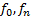)

The frequency of the free oscillations of a system

46 of 47

## At resonance...

• the natural frequency of the forced oscillator is equal to the driving frequency
• the forced oscillator has maximum amplitude
• the forced oscillator absorbs maximum energy from an external source
• the degree of damping affects both the resonant frequency and the amplitude of the forced oscillator
47 of 47